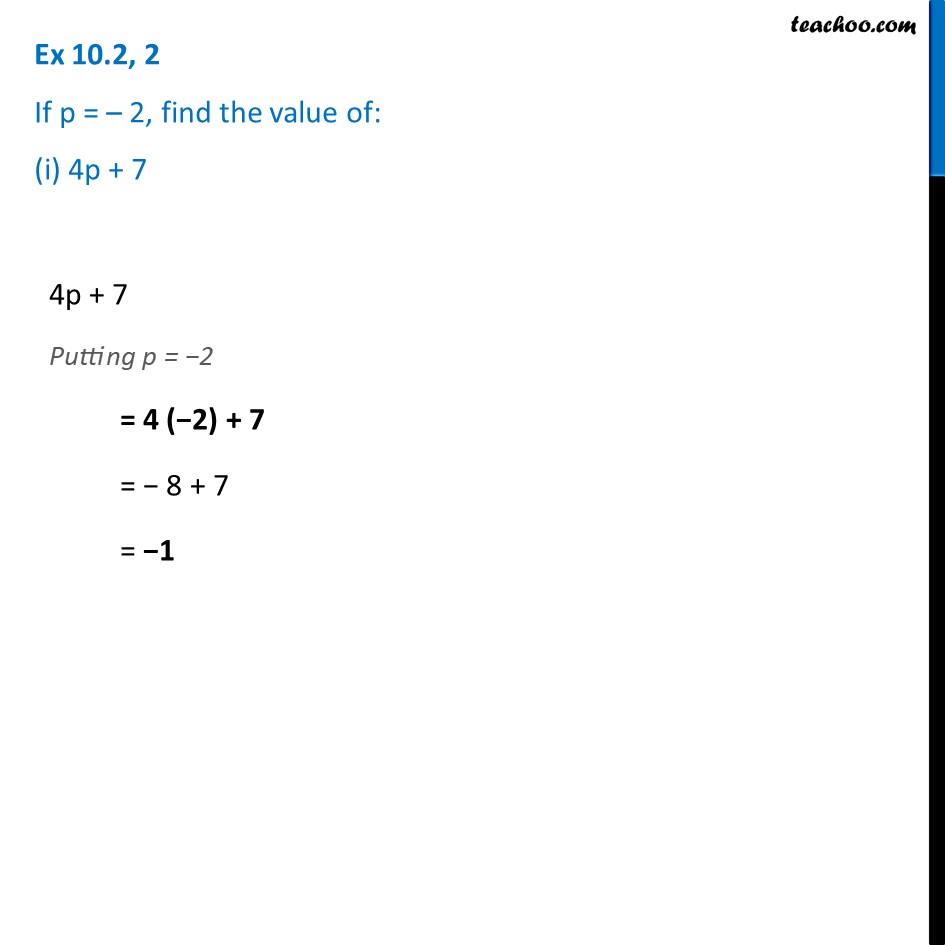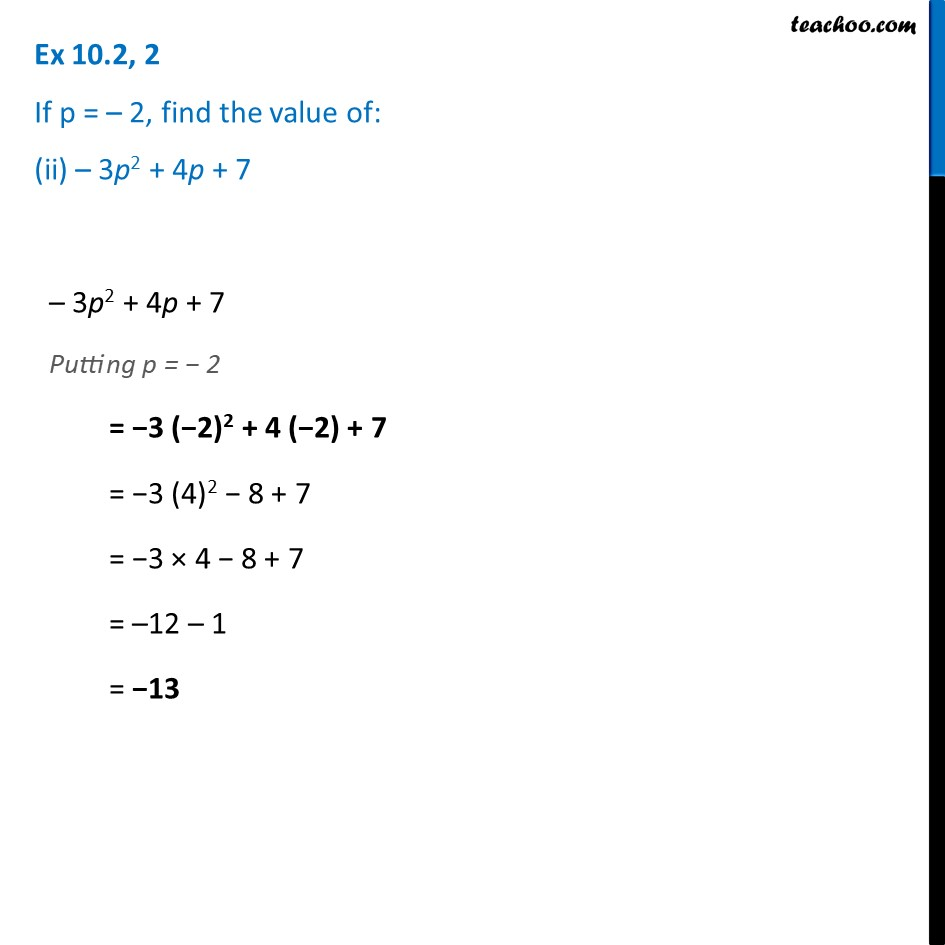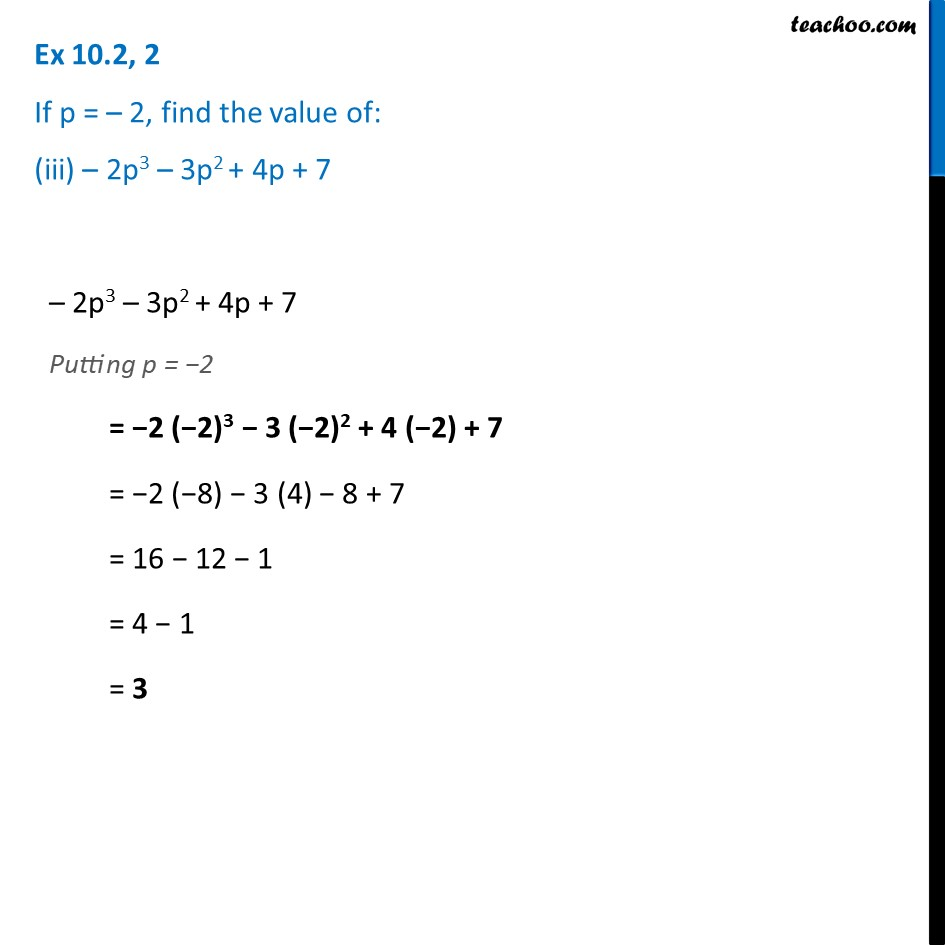Ex 10.2

Chapter 10 Class 7 Algebraic Expressions
Serial order wiseLearn in your speed, with individual attention - Teachoo Maths 1-on-1 Class

### Transcript

Ex 10.2, 2 If p = – 2, find the value of: (i) 4p + 7 4p + 7 Putting p = −2 = 4 (−2) + 7 = − 8 + 7 = −1 Ex 10.2, 2 If p = – 2, find the value of: (ii) – 3p2 + 4p + 7 Ex 10.2, 2 If p = – 2, find the value of: (iii) – 2p3 – 3p2 + 4p + 7 – 2p3 – 3p2 + 4p + 7 Putting p = −2 = −2 (−2)3 − 3 (−2)2 + 4 (−2) + 7 = −2 (−8) − 3 (4) − 8 + 7 = 16 − 12 − 1 = 4 − 1 = 3